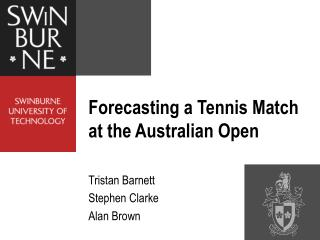Download PresentationForecasting a Tennis Match at the Australian Open

Loading in 2 Seconds...

# Forecasting a Tennis Match at the Australian Open - PowerPoint PPT Presentation

Forecasting a Tennis Match at the Australian Open. Tristan Barnett Stephen Clarke Alan Brown. Introduction. Match Predictions Markov Chain Model Collecting Data Exponential Smoothing Combining Player Statistics Real Time Predictions Combining Sheets from Markov Chain ModelI am the owner, or an agent authorized to act on behalf of the owner, of the copyrighted work described.
Download Presentation## Forecasting a Tennis Match at the Australian Open

An Image/Link below is provided (as is) to download presentation

Download Policy: Content on the Website is provided to you AS IS for your information and personal use and may not be sold / licensed / shared on other websites without getting consent from its author.While downloading, if for some reason you are not able to download a presentation, the publisher may have deleted the file from their server.

- - - - - - - - - - - - - - - - - - - - - - - - - - E N D - - - - - - - - - - - - - - - - - - - - - - - - - -
Presentation Transcript
1. Forecasting a Tennis Match at the Australian Open Tristan Barnett Stephen Clarke Alan Brown

2. Introduction • Match Predictions Markov Chain Model Collecting Data Exponential Smoothing Combining Player Statistics • Real Time Predictions Combining Sheets from Markov Chain Model Bayesian Updating Rule Excel Computer Demonstration

3. Markov Chain Model • Modelling a game of tennis Recurrence Formula: P(a,b) = pP(a+1,b) + (1-p)P(a,b+1) Boundary Conditions: P(a,b) = 1if a=4, b ≤ 2 P(a,b) = 0if b=4, a ≤ 2 where for player A: p = probability of winning a point on serve P(a,b) = conditional probability of winning the game when the score is (a,b)

4. Markov Chain Model Table 1:The conditional probabilities of player A winning the game from various score lines for p = 0.6 • Similarly sheet for player B serving sheets for a set (from sheets of a game) sheet for a match (from sheets of a set)

5. Collecting Data The ATP tour matchfacts: http://www.atptennis.com/en/media/rankings/matchfacts.pdf

6. Collecting Data fi = ai bi + (1 - ai ) ci gi = aav di + ( 1 - aav ) ei where the percentage for player i : fi = points won on serve gi = points won on return ai = 1st serves in play bi = points won on 1st serve ci = points won on 2nd serve di = points won on return of 1st serve ei = points won on return of 2nd serve where the percentage for average player on the ATP tour: aav = 1st serves in play= 58.7%

7. Exponential Smoothing Fit = Fit-1 + [ 1 - ( 1 – α)n ][ fit - Fit-1 ] Git = Git-1 + [ 1 - ( 1 – α)n ] [ git - Git-1 ] where: For player i at period t Fit = smoothed average of the percentage of points won on serve after observing fitGit= smoothed average of the percentage of points won on return of serve after observing git Initialised for average ATP tour player Fi0 = the ATP average of percentage of points won on serve Gi0 = the ATP average of percentage of points won on return of serve n = number of matches played since period t-1 α =smoothing constant • When n=1, [1-(1-α)n] = α, as expected • When n becomes large, [1-(1-α)n] → 1, as expected

8. Combining Player Statistics fij = ft + ( fi - fav ) - ( gj - gav ) • gji = gt + ( gj - gav ) - ( fi - fav ) • where: • For thecombined player statistics • fij= percentage of points won on serve for player i against player j • gji =percentage points won on return for player j against player I • For thetournament averages • ft = percentage of points won on serve • gt = percentage of points won on return of serve • For theATP tour averages • fav = percentage of points won on serve • gav = percentage of points won on return of serve • Since ft + gt = 1, fij + gji = 1 for all i,j as required

9. Combining Sheets The equation for theprobability of player A winning a best-of-5 set match from (e,f) in sets, (c,d) in games, (a,b) in points, player A serving. P''(a,b:c,d:e,f ) = P(a,b) P'B(c+1,d) P''(e+1,f ) + P(a,b) [1-P'B(c+1,d)] P''(e,f+1) + [1-P(a,b)] P'B(c,d+1) P''(e+1,f ) + [1-P(a,b)] [1-P'B(c,d+1)] P''(e,f+1) where for player A : P''(a,b:c,d:e,f ) = probability of winning the match from (a,b:c,d:e,f ) P'B(c,d) = probability of winning the set from (c,d) when player B is serving P''(e,f ) = probability of winning the match from (e,f )

10. Bayesian Updating Rule where: θti= updated percentage of points won on serve at time t for player i μi =initial percentage of points won on serve for player i φti= actual percentage of points won on serve at time t for player i n = number of points played M = expected points to be played When n=0, θ0i= μi as expected When M →0,θti→ φti

11. Computer Demonstration ISF3.XLS 2003 Australian Open Quarter Final El Aynaoui versus Roddick

12. Computer Demonstration ISF4.XLS End of 1st set where: = game to El Aynaoui = game to Roddick = set to El Aynaoui

13. Computer Demonstration End of match where: = game to El Aynaoui by breaking serve = game to Roddick by breaking serve = set to El Aynaoui = set to Roddick